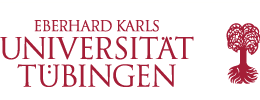Vorträge

Vorträge diese Woche

Stephen Lynch (Universität Tübingen)

We will discuss a geometric flow of compact embedded three-convex hyper- surfaces, where the normal speed at each point of the solution is given by a function of the second fundamental form. Three-convexity means that at each point, the sum of the smallest three principal curvatures is positive. This prop- erty is preserved by the flow if the speed is a concave function which vanishes on the boundary of the cone of three-convex second fundamental forms. For any such flow, the curvature of compact solutions becomes unbounded in finite time, and it is natural to ask whether solutions satisfy a convexity estimate, which implies that high-curvature regions are approximately convex. Convexity esti- mates are known to hold for mean-convex mean curvature flow, and for a large class of fully nonlinear flows of two-convex hypersurfaces. In the three-convex case, however, the concavity of the speed function is a serious impediment to establishing this kind of result. We will introduce here a new speed which, in a sense, strikes a balance between being concave and convex, and thus allows us to overcome this difficulty. The construction is quite general, and can be adapted to the k-convex setting.

 Uhrzeit: 14:15 Ort: C9A03 Gruppe: Oberseminar Geometrische Analysis, Differentialgeometrie und Relativitätstheorie Einladender: Cederbaum, Huisken

Prof. Dr. Peter Kloeden

 Uhrzeit: 17:15 - 18:15 Ort: N14 Gruppe: Kolloquium Einladender: Andreas Prohl

T.B.A.

 Uhrzeit: 16:00 - 17:30 Ort: N 14 Gruppe: Kolloquium Einladender: Felgner, Loose

Dr. Panagiotis Konstantis (Universität Stuttgart)

 Uhrzeit: 17:00 Ort: N 14 Gruppe: Kolloquium Einladender: Loose

Prof. Dr. Bernd Sturmfels (MPI Leipzig)

x-x-Kolloquium mit anschließendem Sommerfest

 Uhrzeit: 15:00 Ort: N14 Gruppe: Kolloquium Einladender: Hannah Markwig